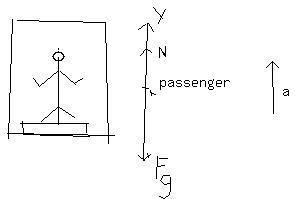# A person stands on a scale inside an elevator at rest. The Scale reads 800N. a) What is the person's mass? (b) The elevator accelerates upward momentarily scale read then? (c) The elevator then moves with a steady speed of 5m/s. What Does the scale read?Anish Buchanan 2021-03-09 Answered
A person stands on a scale inside an elevator at rest. The Scale reads 800N.
a) What is the persons
You can still ask an expert for help

• Questions are typically answered in as fast as 30 minutes

Solve your problem for the price of one coffee

• Math expert for every subject
• Pay only if we can solve itKhribechy

mass m=$\frac{W}{g}$
= weight/acceleration due togravityKey idea is the scale reading is equal to the magnitude of normal force N on the passenger from the scale.
$N-{F}_{g}=ma$
$N={F}_{g}+ma$
This tells us that the scale reading , which is equal to N depends on the vertical acceleration a of the cab. Substituting mg for Fg gives us N = mg +ma = m (g+a)
For any choice of acceleration. a. if it accelerates up the weight will be more because a +ve
if it accelerates downward then weight will decrease because a is - ve then.
If moving with constant speed a =0 so the weight will remain the same as 800N
When suddenly accelates up let us say acceleration = a
Then scale reads m(g + a) = 81.83( 9.8 +a)
When it is moving with constant speed means not moving with any additional acceleration . a = 0
Scale reading = mg = 800N